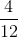## Example Questions

### Example Question #1 : Decimals With Fractions

Find answer in simplified fraction form: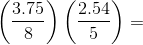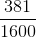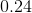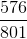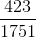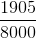Explanation:Multiply both the numerators or denominators by 100 to eliminate the decimals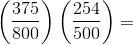Simplify both fractions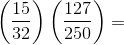Multiply the numerators and multiply the denominators and simplify the fraction by dividing both the numerator and denominator by 5.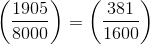### Example Question #1 : How To Find The Decimal Equivalent Of A Fraction

If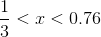can equal which of the following?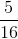None of the other answer choices are correct